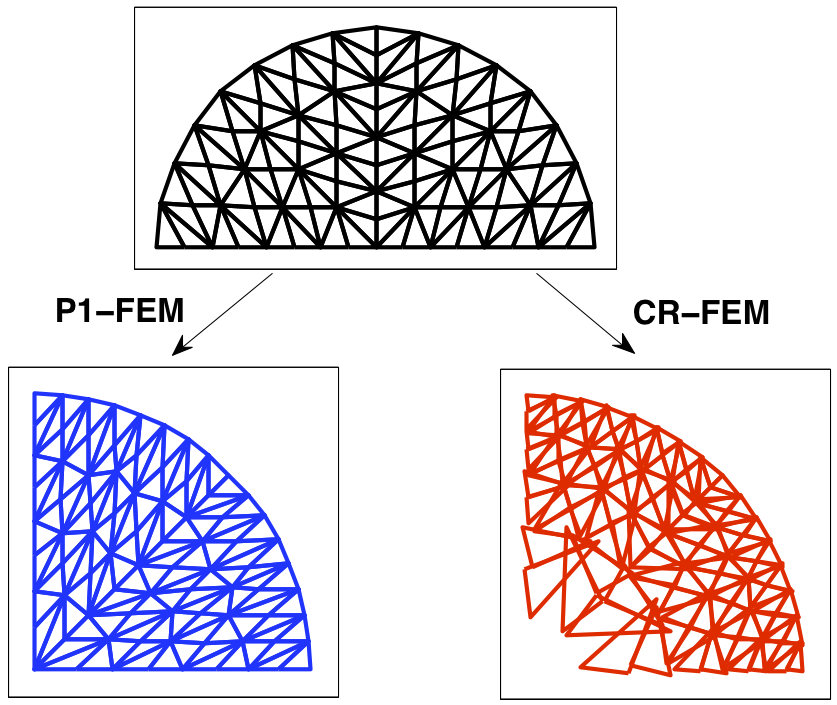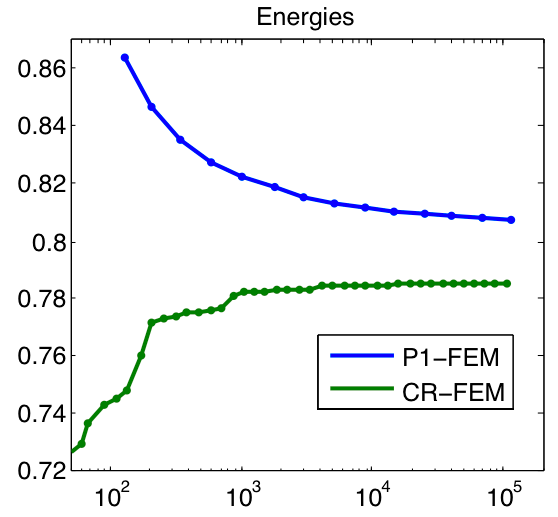Christoph Ortner

In the past I have worked on the numerical approximation of nonlinear elasticity, understood as an energy minimisation problem,

$\bar{u} \in \arg\min\big\{ I[u] \big| u \in A \big\}, \qquad \text{where} \qquad I[u] = \int_\Omega W(x, u , \nabla u)\,dx$

and $A$ is some admissible set. A major difficulty in this area is the Lavrentiev gap phenomenon which is said to occur if

$\inf \big\{ I[u] \big| A \cap W^{1,\infty} \big\} \not\geq \inf \big\{ I[u] \big| u \in A \big\}.$

That is, for certain singular variational problems the infimum of the energy taken over Lipschitz functions can be strictly larger than the infimum taken over the entire admissible class. This means, in particular, that conforming finite element methods are incapable of detecting the global minimizers. I have shown ,  that at least for convex problems (unfortunately this excludes elasticity) one can overcome this by using the non-conforming Crouzeix-Raviart finite element space.

The following figure shows a problem proposed by Foss, Hrusa and Mizel, solved by (mesh-adaptive versions of) the conforming P1 finite element method and the non-conforming Crouzeix-Raviart finite element methods. The left graph clearly shows a gap between the minimal energy (plotted against number of degrees of freedom in the FEM mesh).Although I have not worked on this topic for some time, there is a particularly difficult open problem which I would like to solve, or see solved: to construct a numerical method that is capable (in principle) of approximating global minimizers for any well-posed variational problem (e.g., fitting within the [Ball, 1977] theory) with polyconvex stored energy function.